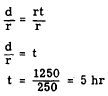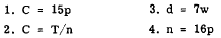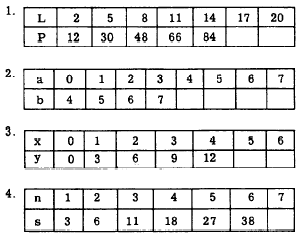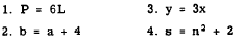Custom SearchEVALUATING FORMULAS The first step in finding the value of the unknown variable of a formula is usually the derivation of a formula that has the unknown as its subject. Once this is accomplished, the evaluation of a formula consists of nothing more than substituting numerical values for the letters representing known quantities and performing the indicated operations. For example, suppose we wish to find the time required to fly 1,250 nautical miles at the rate of 250 knots. The formula is d = rt. We can change the subject by dividing both sides of the equation by r, as follows:Formulas can be solved for an unknown by substituting directly in the original formula even though that unknown is not the subject. Generally, however, it is simpler to first make the unknown the subject. Formulas vary widely, from the simple type such as we have been considering to some that are very complex. All formulas have certain characteristics in common. There is always a subject, the quantity whose value is sought as a final answer. This subject usually stands alone, being placed equal to at least one and possibly several literal numbers, which are combined according to certain indicated operations. The formula can always be evaluated for a specific case when numerical values are known for all these literal quantities. Evaluating formulas may be facilitated by developing a routine order of doing the work. If someone else can read the work and clearly understand what has been done, the work is in good order. The original formula should be written first, then the derived formula that is going to be used in solving the problem, and finally the actual substitutions. The indicated operations may then be carried out. Care should be taken to label answers with correct units; that is, miles per hour, foot-pounds, square feet, etc. Practice problems. 1. E = IR Solve for R in ohms if E is 110 volts and I is 5 amperes. 2. d = rt. Solve for t in hours if d is 840 nautical miles and r is 25 knots. 3.. Solve for C if F is 32�. Answers: 1. 22 ohms  2. 33.6 hr  3. 0� DEVELOPING FORMULAS Developing a formula from a verbal statement is nothing more than reducing the statement to a shorthand form and showing the mathematical relationships between the elements of the statement. For example, suppose that we wish to develop a formula showing the distance, D, traveled at the rate of 20 knots for t hours. If the distance traveled in 1 hr is 20 nautical miles, then the distance traveled in t hours is 20t. Therefore, the formula is D = 20t Practice problems. 1. Write a formula for the cost, C of p pounds of sugar at 15 cents per pound. 2. Write the formula for the cost, C, of one article when the total cost, T, of n similar articles is known. 3. Write a formula for the number of days, d, in w weeks. 4. Write a formula for the number of ounces, n, in p pounds. Answers:Developing Formulas from Tables  In technical work, instrument readings and other data are often recorded in a tabular arrangement. By careful observation of such tables of data, it is frequently possible to find values that are related in a definite pattern. The table can thus be used in developing a formula showing the relationship between the related quantities. For example, table 14-l shows the results of time trials on a ship, with the data rounded to the nearest whole hour and the nearest whole mile. Table 14-1.-Time trials.By inspection of the table, it soon becomes clear that the number of miles traveled is al- ways 20 times the corresponding number of hours. Therefore the formula developed from this table is as follows: d = 20t A second example of the derivation of a formula from a table is shown in figure 14-3. Figure 14-3 (A) shows several polygons (many-sided plane figures), each with one or more diagonals. A diagonal is a straight line joining one vertex (point where two sides meet) with another.Figure 14-3.-Diagonals of plane figures. The table in figure 14-3 (B) compares the number of sides of each polygon with the number of diagonals that can be drawn from any one vertex. Using this table, we make a formula for the number, d, of diagonals that can be drawn from one vertex of a polygon of n sides. In the table we note that the number of diagonals is always 3 less than the number of sides. Therefore the formula is d = n - 3. Practice problems. Complete the following tables and write formulas to show the relationship between the numbers.Answers: